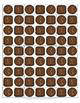digital

# Valentine Candy Math! A Valentine's Day Math Game FreebieSubject
Resource Type
Formats

TpT Digital Activity

PDF (754 KB|6 pages)
Standards
TpT Digital Activity
Add notes & annotations through an interactive layer and assign to students via Google Classroom.
• Product Description
• Standards
Valentine Candy Math! A Valentine's Day Math Game Freebie

This is a 2 player game. Each player takes a game board. 1, 2, or 3 dice are needed for this game. For younger students, roll one die and place the correct number of candies on their heart box. Older students can roll 2 or 3 die and add the numbers together to get the number of candy they should take. When all of the candies are gone, the students count the candy on their game board. The player with the most candy wins. Directions for a subtraction game are also included.

Common Core Standards:
K.CC.1 Count to 100 by ones and tens.
K.CC.4 Understand the relationship between numbers and quantities; connect counting to cardinality.
K.CC.5 Count to answer “how many?” questions about as many as 20 things arranged in a line, a rectangular array, or a circle, or as many as 10 things in a scattered configuration; given a number from 1-20, count out that many objects.
K.CC.6 Identify whether the number of objects in one group is greater than, less than, or equal to the number of objects in another group, e.g./ by using matching and counting strategies. (Include groups with up to 10 objects.)
1.OA.3 Apply properties of operations as strategies to add and subtract.
1.OA.5 Relate counting to addition and subtraction
1.OA.6 Add and subtract within 20, demonstrating fluency for addition and subtraction within 10.

Snowball Fight! A Winter Math Game Freebie
Decorate the Christmas Tree! A Christmas Math Game Freebie
Count the Spiders! A Halloween Math Game Freebie
Get Ready for School! A Back to School Math Game Freebie
Collect the Easter Eggs Game Freebie
St. Patrick's Day Pot O' Gold Game Freebie

Thank you for viewing my Valentine Candy Math! A Valentine's Day Math Game Freebie. I hope you enjoy it!

Carolyn – Exceptional Kinders

Resources
Fonts by: Clip Art by Carrie @ C&C Teach First, www.ccteachfirst.blogspot.com and Kimberly Geswein Fonts, http://www.teacherspayteachers.com/Store/Kimberly-Geswein-Fonts
Graphics by: Cara's Clips, http://www.teacherspayteachers.com/Store/Cara-Taylor-8656
to see state-specific standards (only available in the US).
Add and subtract within 20, demonstrating fluency for addition and subtraction within 10. Use strategies such as counting on; making ten (e.g., 8 + 6 = 8 + 2 + 4 = 10 + 4 = 14); decomposing a number leading to a ten (e.g., 13 - 4 = 13 - 3 - 1 = 10 - 1 = 9); using the relationship between addition and subtraction (e.g., knowing that 8 + 4 = 12, one knows 12 - 8 = 4); and creating equivalent but easier or known sums (e.g., adding 6 + 7 by creating the known equivalent 6 + 6 + 1 = 12 + 1 = 13).
Relate counting to addition and subtraction (e.g., by counting on 2 to add 2).
Apply properties of operations as strategies to add and subtract. If 8 + 3 = 11 is known, then 3 + 8 = 11 is also known. (Commutative property of addition.) To add 2 + 6 + 4, the second two numbers can be added to make a ten, so 2 + 6 + 4 = 2 + 10 = 12. (Associative property of addition.)
Identify whether the number of objects in one group is greater than, less than, or equal to the number of objects in another group, e.g., by using matching and counting strategies.
Count to answer “how many?” questions about as many as 20 things arranged in a line, a rectangular array, or a circle, or as many as 10 things in a scattered configuration; given a number from 1-20, count out that many objects.
Total Pages
6 pages
N/A
Teaching Duration
N/A
Report this Resource to TpT
Reported resources will be reviewed by our team. Report this resource to let us know if this resource violates TpT’s content guidelines.
• Ratings & Reviews
• Q & A

Teachers Pay Teachers is an online marketplace where teachers buy and sell original educational materials.